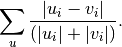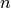# scipy.spatial.distance.canberra¶

scipy.spatial.distance.canberra(u, v)

Computes the Canberra distance between two n-vectors u and v, which is defined asParameters : u : ndarray An-dimensional vector. v : ndarray An-dimensional vector. d : double The Canberra distance between vectors u and v.

Notes

Whe u[i] and v[i] are 0 for given i, then the fraction 0/0 = 0 is used in the calculation.

#### Previous topic

scipy.spatial.distance.braycurtis

#### Next topic

scipy.spatial.distance.chebyshev